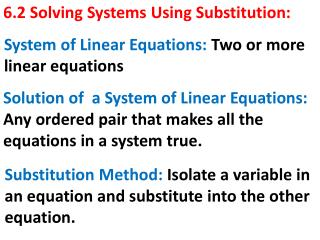DownloadDownload Presentation6.2 Solving Systems Using Substitution:

# 6.2 Solving Systems Using Substitution:

Télécharger la présentation## 6.2 Solving Systems Using Substitution:

- - - - - - - - - - - - - - - - - - - - - - - - - - - E N D - - - - - - - - - - - - - - - - - - - - - - - - - - -
##### Presentation Transcript

1. System of Linear Equations: Two or more linear equations 6.2 Solving Systems Using Substitution: Solution of a System of Linear Equations: Any ordered pair that makes all the equations in a system true. Substitution Method: Isolate a variable in an equation and substitute into the other equation.

2. Remember:

3. GOAL:

4. USING SUBSTITUTION: To solve a system by the substitution method we must: 1) Pick one of the equations and isolate one of the variables. 2) Use isolated variable to substitute on the second equation. 3) Find the value of the variable

5. USING SUBSTITUTION: Continue 4) Substitute back into original equation to obtain the value of the second variable. 5) Check, substitute the values found into the equations to see if the values make the equations TRUE.

6. Ex:What is the solution of the system? Use a graph to check your answer.

7. SOLUTION: 1) Isolate a variable. Notice that on the second equation the y is already isolated. 2) Use isolated variable to substitute on the second equation.

8. SOLUTION: 3) Find the value of the variable.

9. SOLUTION: 4) Substitute back into original equation to obtain the value of the second variable. OR Solution to the problem in ordered pair is: (-2, 0).

10. SOLUTION: 5) Check: substitute the variables to see if the equations are TRUE. and

11. YOU TRY IT:What is the solution of the system? Use substitution.

12. SOLUTION: 1) Isolate a variable. Notice that on the second equation the y is already isolated. 2) Use isolated variable to substitute on the second equation.

13. SOLUTION: 3) Find the value of the variable.

14. SOLUTION: 4) Substitute back into original equation to obtain the value of the second variable. OR Solution to the problem in ordered pair is: (-8, -9).

15. SOLUTION: 5) Check: substitute the variables to see if the equations are TRUE. and